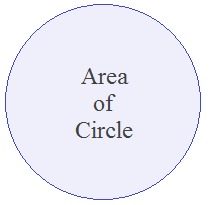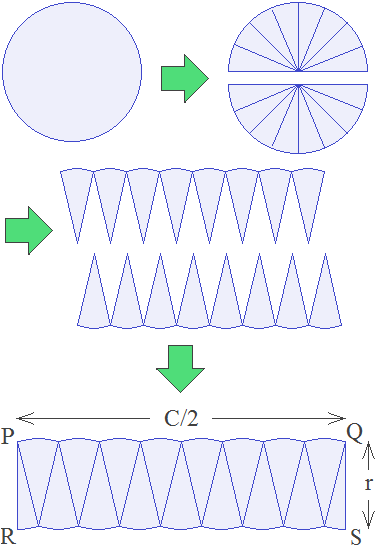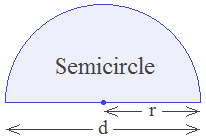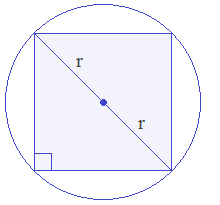# Area of Circle## What is Area of Circle?

The Area of Circle Definition: “Total surface on the plane covered by the circumference of a circle is called the Area of Circle.”

**********************

10 Math Problems officially announces the release of Quick Math Solver, an android APP on the Google Play Store for students around the world.**********************How to find area of circle?

Area of circle can be calculated by using the formula Area = πr2 or πd2/4, where r is the radius of circle and d is the diameter of circle. This formula can be derived from the following activities:1.    Take a circular-shaped paper.

2.    Cut the circle into two equal halves.

3.    Cut each half further into small pieces equally as you can see in the figure.

4.    Arrange the pieces of two halves in one place to form like a rectangle as shown in the figure.

5.    As we go on increasing the number of small pieces it will tend to be a rectangle PQRS of length = half of circumference (c/2) and breadth = radius (r).

We know,

Circumference of the circle (c) = 2πr

Length of rectangle (l) = C/2 = 2πr/2 = πr

Breadth of rectangle (b) = r

Now,

Area of circle,

= Area of rectangle PQRS

= πr × r

= πr2

Since, the radius is half of the diameter, the area of a circle in terms of diameter can be found by replacing r = d/2, i.e.

Area of circle = π(d/2)2 = πd2/4

Therefore, Area of Circle Formula is given by,

Area of circle = πr2

And, area of a circle with diameter is given by,

Area of circle = πd2/4

#### How to find the area of a circle with the circumference?

If the circumference of a circle is given, we have to find first the radius or diameter of the circle from the given circumference. Then, we can calculate the area of a circle by using the formula in terms of radius or diameter.

#### How to find the radius of a circle with the area?

We have,

πr2 = A (Area of the circle)

i.e.    r2 = A/π

or,     r = √(A/π)

Therefore, the radius of circle formula with the area is given by,

r = √(A/π)

How to find the diameter of a circle with the area?

We have,

πd2/4= A (Area of the circle)

i.e.    πd2 = 4A

or,     d2 = 4A/π

or,     d = √(4A/π)

Therefore, the diameter of the circle formula with the area is given by,

d = √(4A/π)

### Semicircle | What is a Semicircle?Semicircle is half of a circle. The diameter of a circle divides a circle into two equal parts, and each part is called a semicircle.

#### Area of Semicircle

Since semicircle is exactly the half of a full circle. Its area is given by the half of the area of full circle. Therefore, the area of semicircle formula is given by,

Area of Semicircle =  πr2/2

### Sector | What is sector?The region enclosed by any two radii and the arc of a circle is called a Sector of the circle. In the figure, the shaded region POQ is an example of a sector. There are minor sector and major sector. The shaded region is a minor sector and the unshaded region is a major sector.

#### Area of sector | How to find the area of a sector?The area of a sector can be calculated by the area of the full circle and the central angle made by the corresponding sector. Since a complete central angle in a circle is 360°, each angle represents the 1/360 part of the area of a full circle. If r is the radius of circle and θ° is the central angle made by a minor sector, then the area of sector formula is given by the following formula,

Area of minor sector = πr2θ/360°

Area of major sector = πr2(1 - θ/360°)

### Worked Out Examples

Example 1: find the area of a circle of radius 14 cm?

Solution: Here,

We know,

Area of circle = πr2

= 22/7 × (14cm)2

= 22/7 × 196 cm2

= 616cm2

Area of circle is 616cm2. Ans.

Example 2: what is the area of a circle with a diameter of 10cm?

Solution: Here,

Diameter (d) = 10 cm

Radius (r) = d/2 = 10/2 = 5cm

We know,

Area of circle = πr2

= 22/7 × 52

= 22/7 × 25

= 78.6cm2

Area of circle is 78.6cm2. Ans.

Example 3: Find the area of circle whose circumference is 44 cm.

Solution: Here,

Circumference (C) = 44 cm

i.e.    2πr = 44

or,     2 × 22/7 × r = 44

or,     44/7 × r = 44

or,     r = 44 × 7/44

or,     r = 7 cm

We know,

Area of circle = πr2

= 22/7 × 72

= 22/7 × 49

= 154cm2

The area of the circle is 154cm2. Ans.

Example 4: Find the circumference of a circle if the area is 300 square centimeters.

Solution: Here,

Area of circle (A) = 300 cm2

i.e.    πr2 = 300

or,     22/7 × r2 = 300

or,     r2 = 300 × 7/22

or,     r2 = 95.45

or,     r2 = 72

or,     r = 9.77 cm

Now,

Circumference of circle = 2πr

= 2 × 22/7 × 9.77

= 61.41 cm

The circumference of the circle is 61.41cm. Ans.

Example 5: If the perimeter and the area of a circle are numerically equal then find the radius of the circle.

Solution: Here,

Perimeter of circle = 2πr

Area of circle = πr2

Perimeter and area of a circle are numerically equal,

πr2 = 2πr

Or,    r = 2 units. Ans.

Example 6: Find the area of the shaded region of the figure.Solution: Here,

Side of square (l) = 8 cm

Area of square (A1) = l2

= (8 cm)2

= 64 cm2

Radius of quarter circle (r) = 8/2 = 4 cm

Area of 4 quarter circles (A2)

= Area of one full circle

= πr2

= 22/7 × 42

= 50.28 cm2

Area of shaded region (A) =  A1 – A2

= 64 cm2 – 50.28 cm2

= 13.72 cm2

Area of the shaded region is13.72 cm2. Ans.

Example 7: Find the area of the shaded region of the figure.Solution: Here,

Length of rectangle (l) = 14 cm

Breadth of rectangle (b) = 7 cm

Area of rectangle (A1) = l × b

= 14 cm × 7 cm

= 98 cm2

Radius of each half circle (r) = 7/2 = 3.5 cm

Area of 4 half circles (A2)

= Area of two full circle

= 2 × πr2

= 2 × 22/7 × (3.5)2

= 77 cm2

Area of shaded region (A) =  A1 – A2

= 98 cm2 – 77 cm2

= 21 cm2

The area of the shaded region is 21cm2. Ans.

Example 8: ABC is a quadrant of a circle of radius 14cm. Find its area.Solution: Here,

We know,

Area of circle = πr2

= 22/7 × (14cm)2

= 22/7 × 196 cm2

= 616cm2

Area of quadrant circle = ¼ of Area of circle

= ¼ × 616cm2

= 154cm2 Ans.

Example 9: Find the area of a square inscribed in a circle with radius r.

Solution: Here,Diameter = 2r

The diameter is the diagonal of the square inscribed in a circle. And, the area of square in terms of diagonal is given by d2/2. Therefore,

Area of the square inscribed in a circle = d2/2

= (2r)2/2

= 4r2/2

= 2r2

Therefore, the area of a square inscribed in a circle is given by the formula,

Area of square = 2r2 Ans.

Example 10: What is the area of the largest square that can be inscribed in a circle of radius 12cm.

Solution: Here,

We know,

Area of the square inscribed in a circle = 2r2

= 2 × (12cm)2

= 2 × 144cm2

= 288cm2 Ans.

If you have any questions or problems regarding the Area of Circle, you can ask here, in the comment section below.